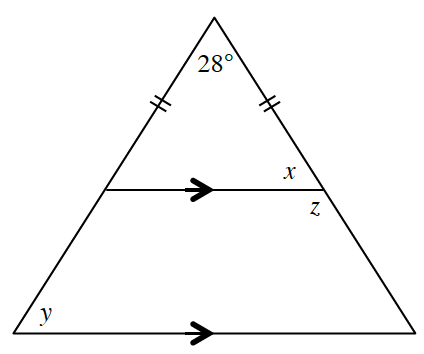### Home > INT2 > Chapter 5 > Lesson 5.1.4 > Problem5-47

5-47.

Determine the measures of $x$$y$ and $z$ at right. Justify each conclusion using geometric relationships.

Remember that the base angles of an isosceles triangle are congruent.
Start by determining the value of $x$.